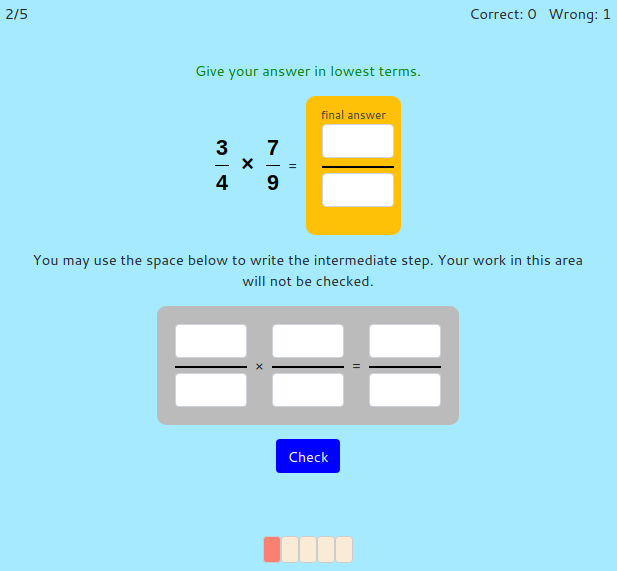MM Practice
×
Multiplication
Division
Place Value
Fractions & Decimals
Measurement
Statistics & Probability
Pre-Algebra
Money
Kindergarten
Geometry

# Multiply Fractions and Mixed NumbersOnline practice for grades 4-7

On this page, you can practice multiplication of fractions and of mixed numbers. You can choose to multiply fractions by whole numbers, fractions by fractions, fractions by mixed numbers, or mixed numbers by mixed numbers. There are also three difficulty levels to choose from: the easy level uses smaller denominators and smaller whole numbers than the medium and challenge levels.

The options are:

• You can limit the script to use only proper fractions—fractions that are less than 1. With this option, the script will make problems such as 1/4 × 2/5, but will not make problems such as 8/5 × 4/3.
• The last option, when chose, allows or accepts answers to not be in lowest terms. In other words, the script will accept an answer such as 8/10.

Note: ALL answers have to be given as mixed numbers, when possible. In other words, your answer cannot be left as an improper fraction.

Multiply... (select at least one)

Level:

Screenshot:OR
Practice for a set time: min

Allow my comment to be posted on this site